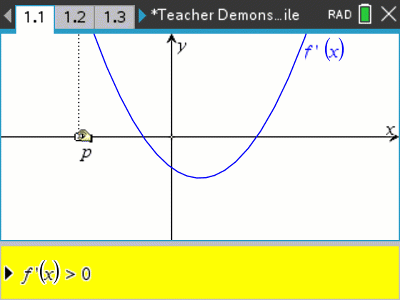# Activities

Graphs of Antiderivatives#### Activity Overview

Students use their TI-Nspire to graph the anti-derivative of a function and investigate aspects of the this function and how it relates to the primitive function. For example, if a continuous derivative function changes from negative to positive, what does this produce on the primitive function? How is that different if the derivative function changed from positive to negative?

#### Objectives

• Deduce the graph of an anti-derivative function from the graph of a given function.

#### Vocabulary

• Derivative
• Primitive function
• Turning point
• Inflexion point
• Stationary point